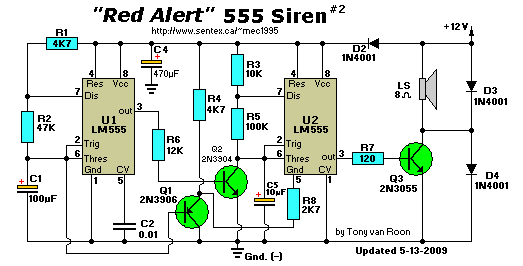```Parts List:
R1,R4 = 4K7                          C1 = 100uF/25V, electrolytic
R2 = 47K                       C2,C3 = 0.01uF (10nF), ceramic
R3 = 10K                          C4 = 470uF/25V, electrolytic
R5 = 100K                         C5 = 10uF/25V, electrolytic
R6 = 12K                     IC1,IC2 = 555 Timer/Oscillator
R7 = 120 ohm                D1,D2,D3 = 1N4001
R8 = 2K7
Q1 = 2N3906                       LS = Loudspeaker, 8 ohm
Q2 = 2N3904
Q3 = 2N3055
```
Notes:
UNDER CONSTRUCTION.

Back to Circuits Page